# Chapter 2b: Strength of acids and bases

The general definition of an acid is thus a compound releasing protons. However, all the acids don’t have the same strength or acidity. We can define two types of acids and bases: Strong acids and bases, and weak acids and bases. For more simplicity, we will focus on acids in this lesson but the principle is identical for bases.

Strong acids

Strong acids totally dissociate in solution. It means that any single molecule of acid put in water will free a proton and acidify the solution. For example, HCl is a strong acid.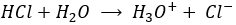If one mole of HCl is put in water, all the HCl dissociates and in solution we can only find H2O, one mole of Cl and one mole of H3O+. For this kind of reaction, the arrow separating reactants and products is a simple arrow going from left to right as the reaction is only going in one way. The potential Hydrogen, or pH, can thus simply be found with the quantity of HCl put in solution. pH=-log [H3O+] and as the reaction is complete, the quantity of H3O+  in solution is equal to the quantity of HCl put in solution. The concentration of protons is thus equal to the concentration of HCl in solution before the reaction [H3O+]=[HCl]0.

For example, if 0.1 mole of HCl is put in water to obtain a total volume of 1l, [H3O+]=0.1mol/l and pH=1. In lab, in general the acid is already in solution with a large concentration (6M for example) and has to be diluted to the desired concentration for the experiment. Remember that precautions are to be taken when you manipulate acids and bases, especially with concentrated ones. Use a pear to pipette them and not your mouth. Another “holy” rule is that “One does not baptise an acid”, meaning that to dilute an acid, add the acid into water and not water into the acid. The reason is that the dilution of an acid is highly exothermic and droplets of acid may be ejected out of the recipient.

The effect of a dilution on the pH is simple. If a solution of pH=2 ([H3O+]=0.01mol/l) is diluted 10 times, the pH increases by one (as it is a logarithmic scale) and pH=3 ([H3O+]=0.001mol/l), etc. For bases, a dilution decreases the pH of the solution towards the neutrality (pH=7). It is indeed the concentration of OH which is affected in this case. As pH=-log[H3O+]=14+log[OH].

Furthermore, a large dilution of an acid won’t lead to a basic solution. The dilution by 100 of a solution of pH=6 does not give a solution of pH=8 but approximately pH=7. In this case the water is the mean species defining the pH. The concentration of the protons coming from the acid becomes negligible with regard to the concentration of protons freed by the water.

To be considered a strong acid, the dissociation constant of the acid has to be large enough to proton all the H2O molecules of the solution into H3O+. Formally, strong acids have a pKa<-1.74. Lets explain that. We have seen that water has a dissociation constant of Kw=10-14mol2l-2. The dissociation constant of an acid is noted Ka. The same way as pH is –log of the proton concentration, pKa=-log Ka. For example HBr has a pKa of -8.7. The limit of pKa<-1.74 is simply the concentration of the water:

In 1l of water there is 1kg of H2O. The molar mass of H2O being equal to 18.01528g, the concentration of pure water is [H2O]=55.5084. –log of this concentration is 1.74.

To resume, to be able to proton all the molecules of water of the solution, which is the condition to be considered a strong acid, the acid has to have a pKa<-1.74. Some acids widely used are usually considered as strong acids but don’t answer to this condition but are between 0>pKa>-1.74 because they fully dissolve in diluted solution. These are the almost strong acids.

Among strong acids, we can find hydrochloric acid (HCl, almost strong acid), sulphuric acid (H2SO4), nitric acid (HNO3, almost strong acid), hydroiodic acid (HI), percloric acid (HClO4), hydrobromic acid (HBr) and many other.

Examples of strong bases: Sodium hydroxide (NaOH), Potassium hydroxide (KOH), Calcium hydroxide (Ca(OH)2),…

The conjugate base of strong acids are very weak bases and are inert as a base. Indeed, the basicity of the conjugate base of an acid (and inversely) is related to the Ka of the acid. The relation is Ka.Kb=Kw=10-14. Imagine for a second that the conjugate base reacts with water. If we add the reactions of the acid and of its conjugate base, we obtain the autoprotolyse of water: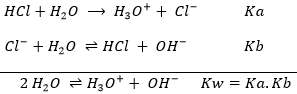HCl has a Ka=103 and the Kb of Cl is thus Kb=10-17.

Weak acids

The acids do not all completely dissolve in water. Acids with pKa>0 are considered as weak acids. Because all of the molecules of acid do not dissolve, there is an equilibrium between the dissociated and the undissociated forms of the acid.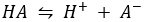The equilibrium is represented by the two arrows between reactants and products.

An example of weak acid is the acetic acid (CH3COOH).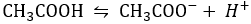In solution, there is thus a melange of those 4 molecules. The pH is still determined by the amount of protons in solution. How do we find this quantity in the case of weak acids?

The constant of reaction of this reaction is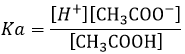Lets take a look at the concentration of the species before and after the reaction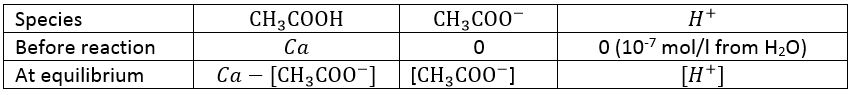One part of the initial concentration of the acid (Ca) has reacted. The quantity of protons and of the conjugate base (CH3COO) produced after the reaction are equal.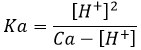Generally, Ca>>[H+] (be carefull with this approximation for diluted solutions), leading to the following relation.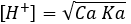The pH of the solution can thus be found from the initial concentration of the acid put in solution and from its Ka:

For bases, the relation is similar:

Those relation can also be written as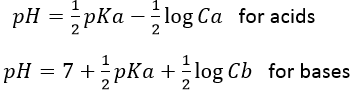From those equations, one can directly see why this kind of acid is weak with regard to strong acids: To obtain an increase of the pH of 1, a strong acid is diluted by 10 while a weak acid has to be diluted by 100.

Contrarily to strong acids, the conjugate bases of weak acids are active as a base in the solution. For example, the conjugate base of a weak acid with Ka=10-4 has a Kb=10-10.

Factors influencing the acidity

Electronegativity: The electronegativity refers to the ability of atoms to keep its electrons and the electrons of the bonds he shares near its nucleus. For two bonded atoms of same electronegativity, electrons composing the bond are not static but they spend an equal time at each end of the bond (statistically). For two bonded atoms of different electronegativity, electrons spend more time in the vicinity of the atom of larger electronegativity. This generates a separation of charges, a dipole with a partial negative charge (noted δ) on the electronegative element and a partial positive charge (noted δ+) on the electropositive element. In acids, H possesses a partial positive charge depending on the electronegativity of the atom he is bonded to.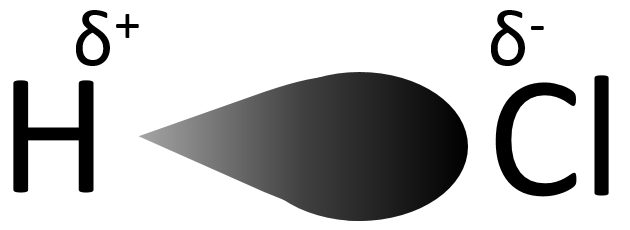This partial positive charge stimulates the dissociation of H and thus increases the acidity of the molecule. Taking a look to the Mendeleev table, moving from left to right across a row on the periodic table elements become more electronegative (excluding the noble gases), and the strength of the acid formed by the element and hydrogen atoms increases accordingly.

Electronegative elements that are not directly bonded to the hydrogen can also pull electrons from the hydrogen. The effect is way smaller but should not be neglected.

Radius: Larger atoms have their bonding electrons further from the nucleus than small atoms. Because of this distance, these electrons are less energetic: there is a smaller interaction with the nucleus and the charge of the nucleus is partially shielded by the electrons of the inner layers. As a consequence, the bond is more easily broken to release a proton if the atom wearing the Hydrogen is large. The sequence of acidity for the halogenous acids shows it clearly. Hydrofluorous acid HF is a weak acid (pKa=3.2) and is less acid than HCl, HBr or HI even if its electronegativity is larger than theirs because it is much smaller (by a factor 2 to 3). The bond between the fluorine and the hydrogen is thus stronger because electrons are close to the nucleus. HI is the largest of the sequence and is also the most acidic halogenous acid with a pKa of -9.3>pKaHBr (-8.7)>pKaHCl (-6.3))>> pKaHF (3.2). Moving down a column on the Mendeleev table, the size of the elements increases and become less electronegative. The size effect tends to dominate the variation of electronegativity and the acidity increases of compounds wearing Hydrogens atoms.

Polyacids

Earlier, we mentioned the sulphuric acid, H2SO4. This acid has two protons available.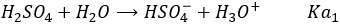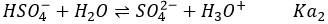H2SO4 is a strong acid (pKa1=-3). When sulphuric acid is put in solution, a first proton is freed and there should be no remaining H2SO4 in solution. On the other hand, HSO4 is a weak acid (pKa2=1.9) and it will not totally dissociate in water. To determine the pH, we can proceed as we did for the weak acid:

With simple math, one can see that [HSO4]= 2Ca- [H3O+] and we can write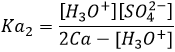From that point, we obtain a second degree equation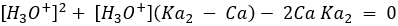That has now to be solved to obtain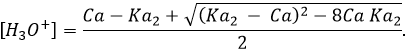The pH can still be calculated from the constant of dissociation and from the initial concentration of acid put in solution even if the solution is a bit more difficult.

Amphoteric species

Amphoteric species are species who show both acidic and basic characteristics. HCO3 is an example of amphoteric species. As for the sulphuric acid, H2CO3 is a polyacid. However the carbonic acid is a weak acid and there is thus an equilibrium involving HCO3 as the conjugate base of H2CO3.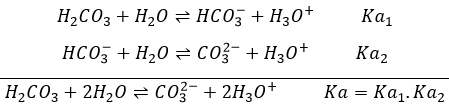Water is also an amphoteric species as it can free or accept a proton.

There is a particular pH at which the amphoteric species has the same effect as a base than as an acid. This pH is called the isoelectric pH or pI. For H2O, the isoelectric pH is 7 but it can be determined from the value of Ka: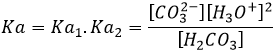Considering that [H2CO3]=[ CO32-] at this pH,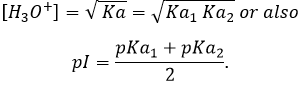Amino acid is another amphoteric species. Such kind of molecule wears an acid group and a basic group. Fig.1 shows the structure of an amino acid in its acidic (left), neutral (middle) and basic form (right). The acid group of the amino acid is the COOH group. Its hydrogen atom can be released to obtain a negative charge on the oxygen. This charge is stabilised by resonance by the COO group. The amine group on the left of the molecule has the role of the base. The azote possess a pair of electrons available to accept a proton.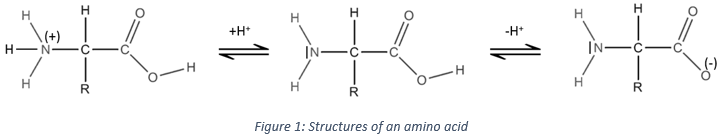This amphoteric property of the amino acid is used experimentally to separate amino acids between them. Because of their particular structure (R varies depending on the amino acid), each amino acid has a different isoelectric pH. The molecules are placed on a gel containing a gradient of pH, inside an electric field. As long as the amino acid is not in its neutral form, it is attracted by an electrode placed at an extremity of the gel. Each amino acid will thus stop to move at a different place on the gel and can be separated. For example Alanine (R=CH3) has a pI=6 while pI=5.48 for the phenylalanine (R=CH2C6H5).

Exercices

You can find here a few exercices to apply the theory explained in this section and eventually sections related to its subject. Most of the questions should be simple to answer but some may require a calculator, or be thougher. Answers are given underneath.

1. What is the pH of a solution of HCl 0.5M?
2. How do I procede experimentally to obtain 100ml of HCl 0.05M?
3. What is the pH of this solution?
4. If I put a droplet of this solution on a bit of pH paper, what color does the paper take?
5. Is HClO (Cl-O-H) an acid or a base?
6. What is the pH of a solution of HClO 0.025M (pKa=7.497)?
7. If this solution is diluted by 10, what is its pH? and if diluted by another 10?
8. What is the pH of a solution of NaOH 0.01M? of NH3 0.01M (Kb=1.8×10−5)? At the equilibrium, how much NH3 remains in solution?

1. pH=0.3: HCl is a strong acid and completely dissociates in solution. pH=-log[H3O+]=-log[HCl]=0.3
2. Even if the acid is not very concentrated in this case, it still has a high pH and precautions have to be taken to manipulate such a solution. Here, we just need to do a dilution of the acid solution by 10. To obtain 100ml of diluted solution, we will need a pipette of 10ml and its pear, and a volumetric flask of 100ml. One does not baptise an acid. The flask is thus first filled with a volume water (50ml for example) and 10ml of the acid solution is added using the pipette and its pear. Avoid to use your mouth. Mix the solution and add water to the graduation. Mix one more time.
3. pH=1.3: As the initial solution has been diluted by 10, pH increases by 1. No need to calculate here.
4. Deep red
5. HClO is an acid: it was fully explained in the previous section: in HClO (Cl-O-H), the electronegativity difference between Cl-O is larger than the one between O-H. The hypochlorous acid splits in ClO and H+. χCl=3.16, χH=2.2, χO=3.44
6. pH=4.55. The hypochlorous acid is a weak acid. The pH formula is then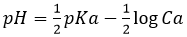7. pH=5.05 for a dilution by 10 and 5.55 for a dilution by 100.
8. NaOH: pH=12, NH3: pH=10.63, [NH3]=0.009576M. We can here simply use the formula of weak bases for NH3 (remember that the pKa+pKb=pKe) but we will need the details for the third part of the question, so lets develop the problem: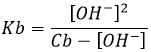Considering that Cb>>[OH], and we will see that this approximation is correct, we find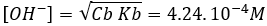So from the 0.01M of NH3 that were put in solution, only ~4% dissociate. The remaining concentration of NH3 is [NH3]=0.009576M. pH is found using pH=14+pOH=10.63.